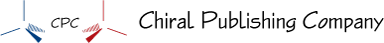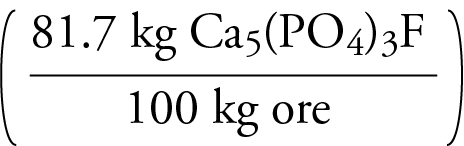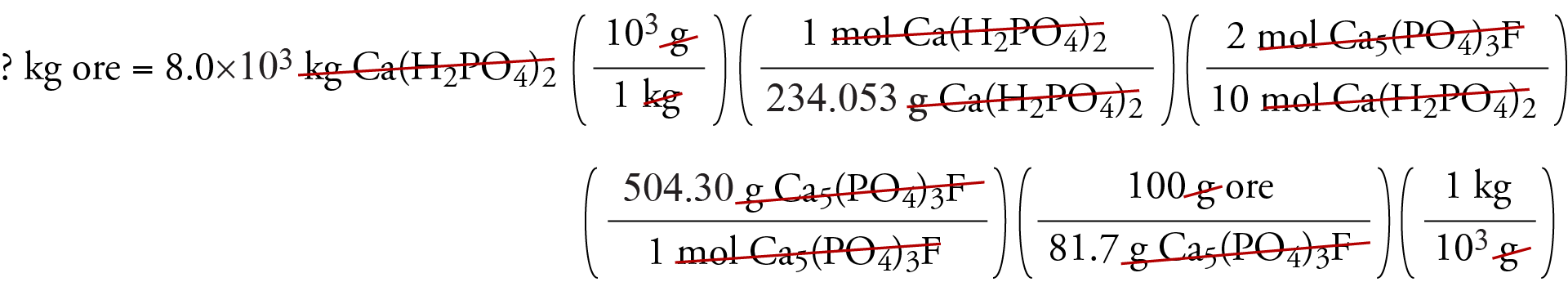﻿ Stoichiometry and Mixtures

#Equation Stoichiometry and Mixtures

• In many equation stoichiometry problems, we can assume that the liquids and solids in our reaction mixtures were pure. The real world is physically quite complex, and chemists must often work with substances that are part of a mixture. For example, apatite, Ca5(PO4)3F, which is a common reactant used to make a number of important compounds, is often added to the reaction vessel in the form of apatite ore, which is a mixture of Ca5(PO4)3F with other substances. The amount of Ca5(PO4)3F in the ore is usually described in terms of percentage. A typical apatite ore has over 75% Ca5(PO4)3F.

Example - Equation Stoichiometry with Mixtures

Calcium dihydrogen phosphate, Ca(H2PO4)2, which is used in the production of triple superphosphate fertilizers, can be formed from the reaction of phosphoric acid with apatite, Ca5(PO4)3F, in apatite ore.

2Ca5(PO4)3F  +  14H3PO4        10Ca(H2PO4)2  +  2HF

a.  What is the maximum mass of Ca(H2PO4)2 that can be formed from the reaction of excess phosphoric acid solution with the Ca5(PO4)3F in 8.50×104 kilograms of an apatite ore that is 81.7% Ca5(PO4)3F?

b.  If an apatite ore is 81.7% Ca5(PO4)3F, what is the minimum mass necessary to produce 8.0×103 kg of Ca(H2PO4)2?

Solution:

a.  Percentages convey a ratio that can be used as a conversion factor. Thus 81.7% Ca5(PO4)3F in apatite ore can be described asThis conversion factor can be used to convert between mass of ore and mass of the pure substance Ca5(PO4)3F. The following shows one way to work this problem.= 1.61 x 105  kg Ca(H2PO4)2

b. For this calculation, the percentage provides a conversion factor that converts from mass of
Ca5(PO4)3F to mass of the apatite ore.= 4.2 x 103 kg ore

Chiral Publishing Company